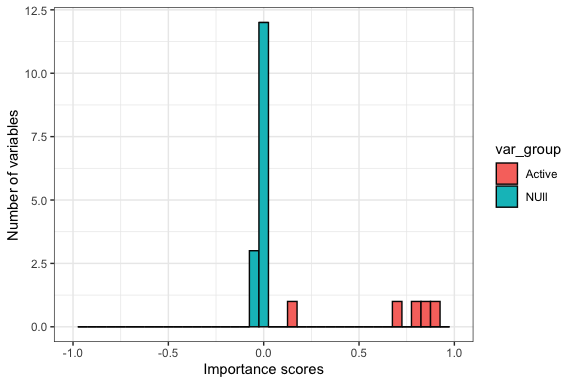# Vignette of R package kko

This package provides a kernel knockoffs selection procedure, dubbed KKO, for the nonparametric additive model. The procedure integrates three key components: the knockoffs, the subsampling for stability, and the random feature mapping for nonparametric function approximation. Finite-sample false discovery rate (FDR) control guarantee is established for KKO, see Dai et al. (2021).

# Generate data

Let us begin by creating some synthetic data. The data is generated from additive polynomial function.

library(ggplot2)
library(kko)
library(knockoff)
set.seed(12345)

### generate regression coefficent
p=20 # number of predictors
sig_mag=10 # signal strength
s=5  # sparsity, number of nonzero component functions
reg_coef=c(rep(1,s),rep(0,p-s))  # regression coefficient
reg_coef=reg_coef*(2*(rnorm(p)>0)-1)*sig_mag

### generate response and design
model="poly"
n= 600 # sample size
X=matrix(rnorm(n*p),n,p)   # generate design
X_k = create.second_order(X) # generate knockoff
y=generate_data(X,reg_coef,model) # response

# Kernel knockoffs selection

We then apply KKO method to generate importance scores of variables.

rkernel="laplacian" # kernel choice
rk_scale=1  # scaling paramtere of kernel
rfn_range=c(2,3,4)  # number of random features
cv_folds=15  # folds of cross-validation in group lasso
n_stb=200 # number of subsampling for importance scores
n_stb_tune=100 # number of subsampling for tuning random feature number
frac_stb=1/2 # fraction of subsample
nCores_para=2 # number of cores for parallelization

### KKO selection
kko_fit=kko(X,y,X_k,rfn_range,n_stb_tune,n_stb,cv_folds,frac_stb,nCores_para,rkernel,rk_scale)

The importance scores by KKO are the difference of selection frequencies between variables and knockoffs, ranging from $$-1$$ to $$1$$. The active variables are expected to have high positive scores (close to one). Those of null variables are expcted to stay centered at zero.

reg_coef  # true regression coefficient 
##    10  10 -10 -10  10   0   0   0   0   0   0   0   0   0   0   0   0   0   0
##    0
W=kko_fit\$importance_score # knockoff importance scores generated by KKO
W 
##    0.703333333  0.160000000  0.870000000  0.886666667  0.776666667
##   -0.006666667  0.023333333 -0.040000000 -0.006666667  0.000000000
##  -0.003333333 -0.003333333 -0.003333333  0.000000000 -0.043333333
##  -0.016666667 -0.030000000  0.003333333  0.000000000 -0.003333333
mydata=data.frame(W=W,var_group=ifelse(reg_coef!=0,"Active","NUll"))
myplot = ggplot(mydata, aes(W, fill = var_group)) +
geom_histogram(color = "gray2",binwidth=1/p) + theme_bw()+
xlab("Importance scores")+ylab("Number of variables")+
xlim(-1,1)

print(myplot)
## Warning: Removed 4 rows containing missing values (geom_bar).# Knockoff filtering

We apply knockoff filter on KKO importance scores. The filter computes a threshold on scores, and pick significant variables above the threshold.

fdr=0.2 #FDR control level
thres = knockoff.threshold(W, fdr=fdr) # thresholding on scores by knockoff filter
selected = which(W >= thres)
selected  # indices of selected variables 
##  1 2 3 4 5

# Reference

1. Xiaowu Dai, Xiang Lyu, and Lexin Li. Kernel Knockoffs Selection for Nonparametric Additive Models. arXiv preprint arXiv:2105.11659 (2021).